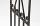# Equilateral triangle

How long should be the minimum radius of the circular plate to be cut equilateral triangle with side 19 cm from it?

Result

r =  10.97 cm

#### Solution:Leave us a comment of example and its solution (i.e. if it is still somewhat unclear...):

Showing 0 comments:Be the first to comment!#### To solve this verbal math problem are needed these knowledge from mathematics:

See also our right triangle calculator. See also our trigonometric triangle calculator.

## Next similar examples:

1. Height 2Calculate the height of the equilateral triangle with side 38.
2. Cable car 2Cable car rises at an angle 41° and connects the upper and lower station with an altitude difference of 1175 m. How long is the track of cable car?
3. SAS triangleThe triangle has two sides long 7 and 19 and included angle 36°. Calculate area of this triangle.
4. 30-60-90The longer leg of a 30°-60°-90° triangle measures 5. What is the length of the shorter leg?
5. n-gonWhat is the side length of the regular 5-gon inscribed in a circle of radius 12 cm?
6. Hexagon 5The distance of parallel sides of regular hexagonal is 61 cm. Calculate the length of the radius of the circle described to this hexagon.
7. RT - inscribed circleIn a rectangular triangle has sides lengths> a = 30cm, b = 12.5cm. The right angle is at the vertex C. Calculate the radius of the inscribed circle.
8. ReflectorCircular reflector throws light cone with a vertex angle 49° and is on 33 m height tower. The axis of the light beam has with the axis of the tower angle 30°. What is the maximum length of the illuminated horizontal plane?
9. Center traverseIt is true that the middle traverse bisects the triangle?
10. SinesIn ▵ ABC, if sin(α)=0.5 and sin(β)=0.6 calculate sin(γ)
11. An angleAn angle x is opposite side AB which is 10, and side AC is 15 which is hypotenuse side in triangle ABC. Calculate angle x.
12. High wallI have a wall 2m high. I need a 15 degree angle (upward) to second wall 4 meters away. How high must the second wall?
13. FlowerbedFlowerbed has the shape of an isosceles obtuse triangle. Arm has a size 5.5 meters and an angle opposite to the base size is 94°. What is the distance from the base to opposite vertex?
14. Inscribed triangleTo a circle is inscribed triangle so that the it's vertexes divide circle into 3 arcs. The length of the arcs are in the ratio 2:3:7. Determine the interior angles of a triangle.
15. Reference angleFind the reference angle of each angle:
16. TrigonometryIs true equality? ?
17. Theorem proveWe want to prove the sentence: If the natural number n is divisible by six, then n is divisible by three. From what assumption we started?